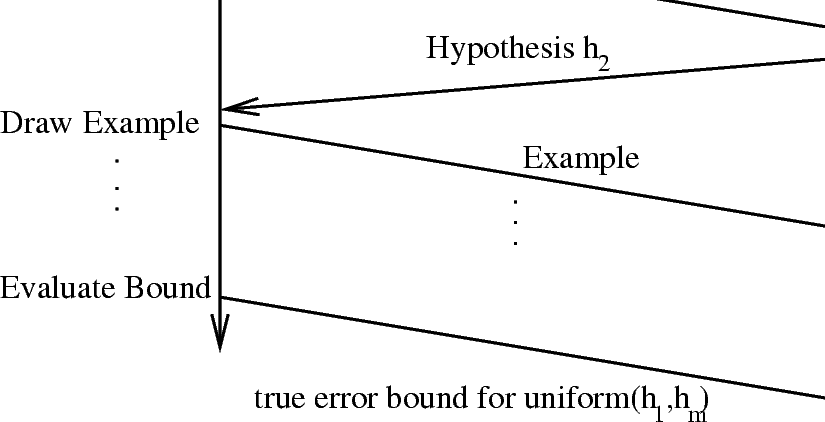### 10.1. Progressive Validation Technique

Progressive validation is a technique which allows you to use almost $\frac{1}{2}$ of the data in a holdout set for training purposes while still providing the same guarantee as the holdout bound. It first appeared in  and is discussed in a more refined and detailed form here.

Suppose that you have a training set of size ${m}_{\text{train}}$ and test set of size ${m}_{\text{pv}}$. Progressive validation starts by first learning a hypothesis on the training set and then testing on the first example of the test set. Then, we train on training set plus the first example of the test set and test on the second example of the test set. The process continues ${m}_{\text{test}}$ iterations. Let $m$ abbreviate ${m}_{\text{pv}}$. Then, we have $m$ hypotheses, ${h}_{1},...,{h}_{m}$ and $m$ error observations, ${\stackrel{̂}{e}}_{1},...,{\stackrel{̂}{e}}_{m}$. The hypothesis output by progressive validation is the randomized hypothesis which chooses uniformly from ${h}_{1},...,{h}_{m}$ and evaluates to get an estimated output. Note that this protocol is similar to those in  and the new thing here is an analysis of performance.

Since we are randomizing over hypotheses trained on ${m}_{\text{train}}$ to ${m}_{\text{train}}+{m}_{\text{pv}}-1$ examples, the expected number of examples used by any hypothesis is ${m}_{\text{train}}+\frac{{m}_{\text{pv}}-1}{2}$. Given that training can exhibit phase transitions, the extra few examples can greatly improve the accuracy of the trained example.

Viewed as an interactive proof of learning, the progressive validation technique follows the protocol of figure 10.1.1.Figure 10.1.1: The progressive validation protocol has a learner repeatedly commit to a hypothesis before it is given a new example. Based upon the test errors, a bound on the true error rate of the metahypothesis which chooses randomly from each of $\left({h}_{1},...,{h}_{m}\right)$ before each evaluation is provided.

The true error rate of this randomized hypothesis will be: ${e}_{\text{pv}}=\frac{1}{{m}_{\text{pv}}}{\sum }_{i=1}^{{m}_{\text{pv}}}e\left({h}_{i}\right)$ where $e\left({h}_{i}\right)={Pr}_{D}\left({h}_{i}\left(x\right)\ne y\right)$ and the empirical error estimate of this randomized hypothesis will be: ${\stackrel{̂}{e}}_{\text{pv}}=\frac{1}{{m}_{\text{pv}}}{\sum }_{i=1}^{{m}_{\text{pv}}}{\stackrel{̂}{e}}_{i}$